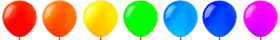# Online math exercises: Exponentiation

52

In sequence level 1 level 2 level 3

Score: Errors:

Choose answer### Online math exercises: Exponentiation

In mathematics, exponentiation (power) is an arithmetic operation on numbers. It can be thought of as repeated multiplication, just as multiplication can be thought of as repeated addition.

You won!

Congratulations